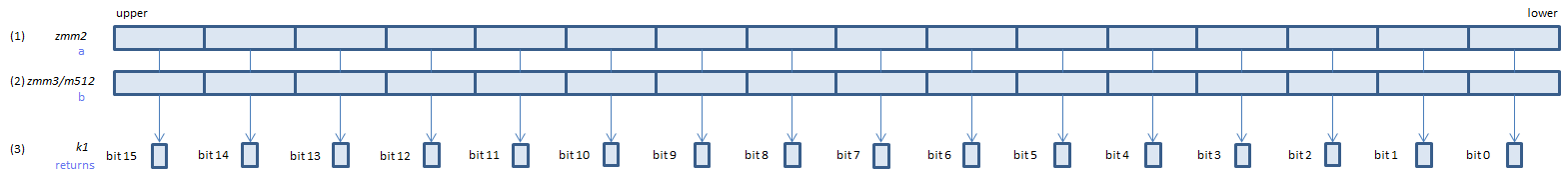﻿ vcmpord_sps

## VCMPORD_SPS - CoMPare ORDered Signal Packed Single

VCMPORD_SPS xmm1, xmm2, xmm3/m128    (V1
__m128 _mm_cmp_ps(__m128 a, __m128 b, _CMP_ORD_S)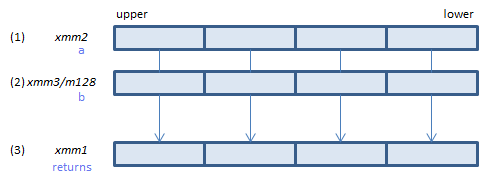For each float, if neither of (1)(2) is NaN, set 1, else set 0, to all bits of the corresponding float of (3).
VCMPORD_SPS ymm1, ymm2, ymm3/m256    (V1
__m256 _mm256_cmp_ps(__m256 a, __m256 b, _CMP_ORD_S)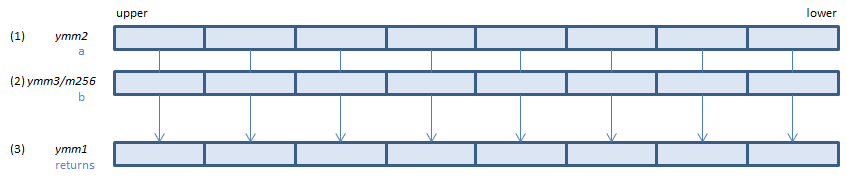For each float, if neither of (1)(2) is NaN, set 1, else set 0, to all bits of the corresponding float of (3).
VCMPORD_SPS k1{k2}, xmm2, xmm3/m128/m32bcst    (V5+VL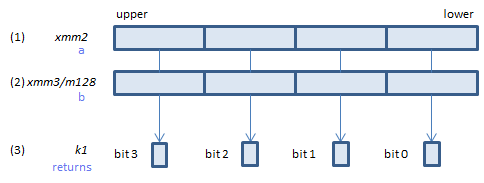For each float, if neither of (1)(2) is NaN, set 1, else set 0, to the corresponding bit of (3).
If k2 bit is 0, the comparison is not done and the corresponding bit of (3) is set to zero. Upper bits of (3) are zero cleared.
VCMPORD_SPS k1{k2}, ymm2, ymm3/m256/m32bcst    (V5+VL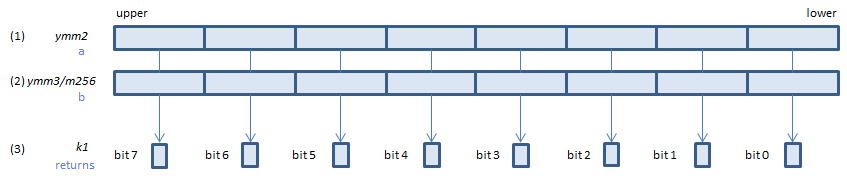For each float, if neither of (1)(2) is NaN, set 1, else set 0, to the corresponding bit of (3).
If k2 bit is 0, the comparison is not done and the corresponding bit of (3) is set to zero. Upper bits of (3) are zero cleared.
VCMPORD_SPS k1{k2}, zmm2, zmm3/m512/m32bcst{sae}    (V5